# Test: The Bipolar Junction Transistor

## 20 Questions MCQ Test RRB JE for Electrical Engineering | Test: The Bipolar Junction Transistor

Description
Attempt Test: The Bipolar Junction Transistor | 20 questions in 60 minutes | Mock test for Electronics and Communication Engineering (ECE) preparation | Free important questions MCQ to study RRB JE for Electrical Engineering for Electronics and Communication Engineering (ECE) Exam | Download free PDF with solutions
QUESTION: 1

### A uniformly doped silicon pnp transistor is biased in the forward-active mode. The doping profile is NE = 108 cm-3, NB = 5.2 x 1016 cm-3 and NC = 1015  cm-3 .For VEB = 0.6 V, the pB at x =0 is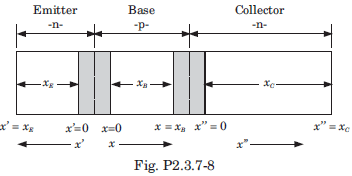Solution: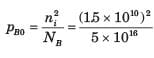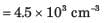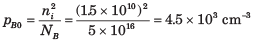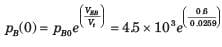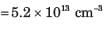QUESTION: 2

### An npn bipolar transistor having uniform doping of NE = 1018 cm-3  NB = 1016 cm-3 and Nc = 6 x 1015 cm-3 is operating in the inverse-active mode with VBE = - 2 V and VBC = 0.6 V. The geometry of transistor is shown in fig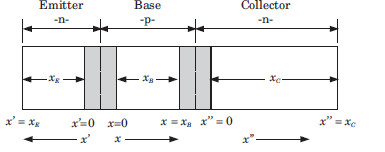Q. The minority carrier concentration at x =  xB is

Solution: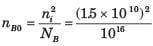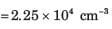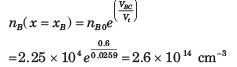QUESTION: 3

### An npn bipolar transistor having uniform doping of NE = 1018 cm-3  NB = 1016 cm-3 and Nc = 6 x 1015 cm-3 is operating in the inverse-active mode with VBE = - 2 V and VBC = 0.6 V. The geometry of transistor is shown in figQ. The minority carrier concentration at x" = 0 is

Solution: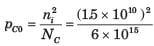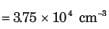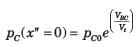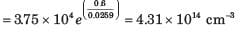QUESTION: 4

An pnp bipolar transistor has uniform doping of NE = 6 x 1017 cm-3, N= 2 x 1016  cm-3 and N= 5 x 1014 cm-3. The transistor is operating is inverse-active mode. The maximum VCB voltage, so that the low injection condition applies, is

Solution:

Low injection limit is reached when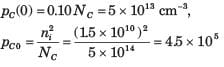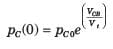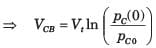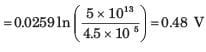QUESTION: 5

The following currents are measured in a uniformly doped npn bipolar transistor:

InE  = 1.20 mA, IpE  = 0.10 mA, InC = 1.18 mA
IR = 0.20 mA, IG = 1 μA, IpC0 = 1 μA

Q.

The α is

Solution: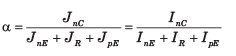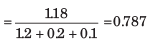QUESTION: 6

The following currents are measured in a uniformly doped npn bipolar transistor:

InE  = 1.20 mA, IpE  = 0.10 mA, InC = 1.18 mA
IR = 0.20 mA, IG = 1 μA, IpC0 = 1 μA

Q.

The β is

Solution: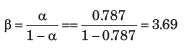QUESTION: 7

The following currents are measured in a uniformly doped npn bipolar transistor:

InE  = 1.20 mA, IpE  = 0.10 mA, InC = 1.18 mA
IR = 0.20 mA, IG = 1 μA, IpC0 = 1 μA

Q.

The  γ is

Solution: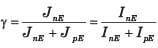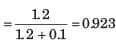QUESTION: 8

A silicon npn bipolar transistor has doping concentration of NE = 2 x 1018cm-3, NB =1017cm-3 and N = 15 x 1016 cm-3. The area is 10-3  cm2 and neutral base width is 1 μm. The transistor is biased in the active region at VBE = 0.5 V. The collector current is
(DB = 20 cm2/s)

Solution: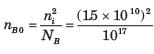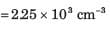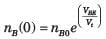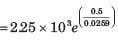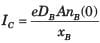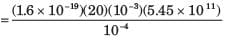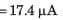QUESTION: 9

A uniformly doped npn bipolar transistor has following parameters:

NE = 1018 cm-3 NB = 5 x 1016 cm-3,
Nc = 2 x 1019 cm-3,
DE = 8 cm2 /s , DB = 15 cm2 /s , Dc = 14 cm2 /s
xE = 0.8 μm, xB = 0.7 μm

The emitter injection efficiency γ is

Solution: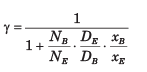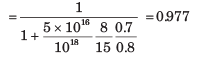QUESTION: 10

In bipolar transistor biased in the forward-active region the base current is IB = 50 μA. and the collector currents is IC = 27 μA. The α is

Solution: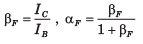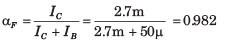QUESTION: 11

For the transistor in fig., IS = 1015 A, βF = 100,  βR = 1. The current ICBO is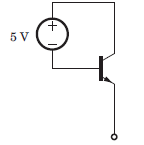Solution: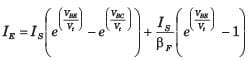= 0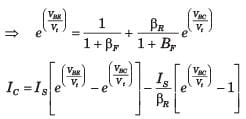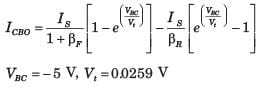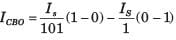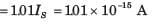QUESTION: 12

Determine the region of operation for the transistor shown in circuit in question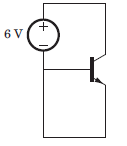Solution: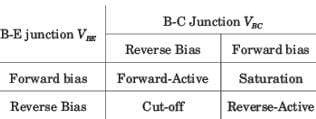VBE = 0 , VBC < 0, Thus both junction are in reverse bias. Hence cut off region.

QUESTION: 13

Determine the region of operation for the transistor shown in circuit in question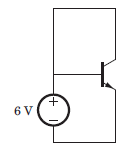Solution:

VBE > 0, VBC = 0, Base-Emitter junction forward bais, Base-collector junction reverse bias, Hence forward-active region.

QUESTION: 14

Determine the region of operation for the transistor shown in circuit in question.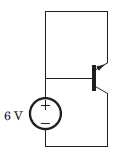Solution:

VBE = 0, VBC> 0, Base-Emitter junction reverse bais , Base-collector junction forward bias, Hence reverse-active region.

QUESTION: 15

Determine the region of operation for the transistor shown in circuit in question.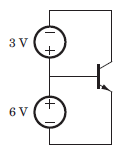Solution:

VBE = 6V, VBC  = 3V Both junction are forward biase, Hence saturation region.

QUESTION: 16

For the circuit shown in fig., let the value of βR =0.5 and  βF = 50. The saturation current is 10-16 A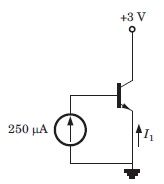Q. The base-emitter voltage is

Solution:

The current source will forward bias the base-emitter junction and the collector base junction will then be reverse biased. Therefore the transistor is in the forward active region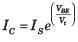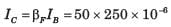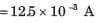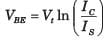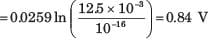QUESTION: 17

For the circuit shown in fig., let the value of βR =0.5 and  βF = 50. The saturation current is 10-16 AQ. The current I1 is

Solution: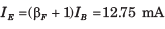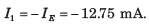QUESTION: 18

The leakage current of a transistor are ICBO = 5μA and ICEO = 0.4 mA, and IB =30 μA

Q. The value of β is

Solution: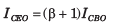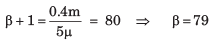QUESTION: 19

The leakage current of a transistor are ICBO = 5μA and ICEO = 0.4 mA, and IB =30 μA

Q. The value of IC is

Solution:

Ic = βIB + ICEO = 79(30μ) + 0.4m = 2.77 mA

QUESTION: 20

For a BJT, IC = 5 mA, IB = 50 μA and ICBO = 0.5μA.

Q. The value of β is

Solution: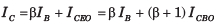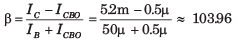Use Code STAYHOME200 and get INR 200 additional OFF Use Coupon Code